# Test: SQL

Test Description

## 30 Questions MCQ Test GATE Computer Science Engineering(CSE) 2023 Mock Test Series | Test: SQL

Test: SQL for Computer Science Engineering (CSE) 2023 is part of GATE Computer Science Engineering(CSE) 2023 Mock Test Series preparation. The Test: SQL questions and answers have been prepared according to the Computer Science Engineering (CSE) exam syllabus.The Test: SQL MCQs are made for Computer Science Engineering (CSE) 2023 Exam. Find important definitions, questions, notes, meanings, examples, exercises, MCQs and online tests for Test: SQL below.
Solutions of Test: SQL questions in English are available as part of our GATE Computer Science Engineering(CSE) 2023 Mock Test Series for Computer Science Engineering (CSE) & Test: SQL solutions in Hindi for GATE Computer Science Engineering(CSE) 2023 Mock Test Series course. Download more important topics, notes, lectures and mock test series for Computer Science Engineering (CSE) Exam by signing up for free. Attempt Test: SQL | 30 questions in 90 minutes | Mock test for Computer Science Engineering (CSE) preparation | Free important questions MCQ to study GATE Computer Science Engineering(CSE) 2023 Mock Test Series for Computer Science Engineering (CSE) Exam | Download free PDF with solutions
 1 Crore+ students have signed up on EduRev. Have you?
Test: SQL - Question 1

### Which of the following statements are TRUE about an SQL query? P : An SQL query can contain a HAVING clause even if it does not have a GROUP BY clause Q : An SQL query can contain a HAVING clause only if it has a GROUP BY clause R : All attributes used in the GROUP BY clause must appear in the SELECT clause S : Not all attributes used in the GROUP BY clause need to appear in the SELECT clause

Detailed Solution for Test: SQL - Question 1

According to standard SQL answer should be C. Refer If we talk about different SQL implementations like MySQL, then option B is also right. But in question they seem to be talking about standard SQL not about implementation. For example below is a P is correct in most of the implementations. HAVING clause can also be used with aggregate function. If we use a HAVING clause without a GROUP BY clause, the HAVING condition applies to all rows that satisfy the search condition. In other words, all rows that satisfy the search condition make up a single group. See this for more details. S is correct . To verify S, try following queries in SQL.

CREATE TABLE temp
(
id INT,
name VARCHAR(100)
);
INSERT INTO temp VALUES (1, "abc"); INSERT INTO temp VALUES (2, "abc"); INSERT INTO temp VALUES (3, "bcd"); INSERT INTO temp VALUES (4, "cde");

SELECT Count(*)
FROM temp
GROUP BY name;

Output:

count(*)
--------
2
1
1

Test: SQL - Question 2

###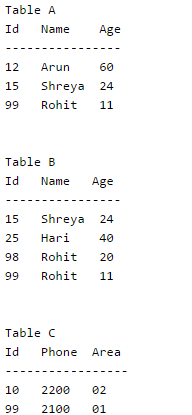Consider the above tables A, B and C. How many tuples does the result of the following SQL query contains?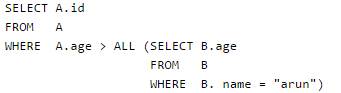Detailed Solution for Test: SQL - Question 2

The meaning of “ALL” is the A.Age should be greater than all the values returned by the subquery. There is no entry with name “arun” in table B. So the subquery will return NULL. If a subquery returns NULL, then the condition becomes true for all rows of A (See this for details). So all rows of table A are selected.

Test: SQL - Question 3

### Consider a database table T containing two columns X and Y each of type integer. After the creation of the table, one record (X=1, Y=1) is inserted in the table. Let MX and My denote the respective maximum values of X and Y among all records in the table at any point in time. Using MX and MY, new records are inserted in the table 128 times with X and Y values being MX+1, 2*MY+1 respectively. It may be noted that each time after the insertion, values of MX and MY change. What will be the output of the following SQL query after the steps mentioned above are carried out? SELECT Y FROM T WHERE X=7;

Test: SQL - Question 4

Database table by name Loan_Records is given below.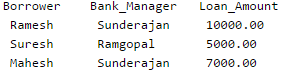What is the output of the following SQL query?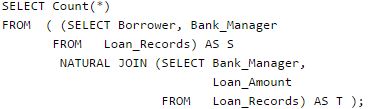Test: SQL - Question 5

A relational schema for a train reservation database is given below. Passenger (pid, pname, age) Reservation (pid, class, tid)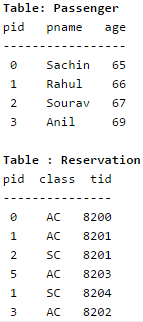What pids are returned by the following SQL query for the above instance of the tables?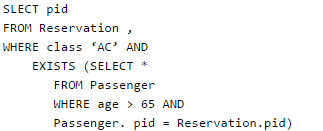Detailed Solution for Test: SQL - Question 5

When a subquery uses values from outer query, the subquery is called correlated subquery. The correlated subquery is evaluated once for each row processed by the outer query. The outer query selects 4 entries (with pids as 0, 1, 5, 3) from Reservation table. Out of these selected entries, the subquery returns Non-Null values only for 1 and 3.

Test: SQL - Question 6

Let R and S be relational schemes such that R={a,b,c} and S={c}. Now consider the following queries on the database: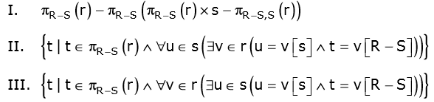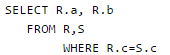Q. Which of the above queries are equivalent?

Detailed Solution for Test: SQL - Question 6

I and II describe the division operator in Relational Algebra and Tuple Relational Calculus respectively. See Page 3 of this and slide numbers 9,10 of this for more details.

Test: SQL - Question 7

Consider the following relational schema:

Suppliers(sid:integer, sname:string, city:string, street:string)
Parts(pid:integer, pname:string, color:string)
Catalog(sid:integer, pid:integer, cost:real)

Consider the following relational query on the above database: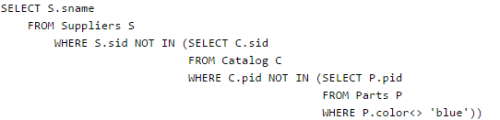Q. Assume that relations corresponding to the above schema are not empty. Which one of the following is the correct interpretation of the above query?

Detailed Solution for Test: SQL - Question 7

The subquery “SELECT P.pid FROM Parts P WHERE P.color<> ‘blue’” gives pids of parts which are not blue. The bigger subquery “SELECT C.sid FROM Catalog C WHERE C.pid NOT IN (SELECT P.pid FROM Parts P WHERE P.color<> ‘blue’)” gives sids of all those suppliers who have supplied blue parts. The complete query gives the names of all suppliers who have supplied a non-blue part

Test: SQL - Question 8

Consider the table employee(empId, name, department, salary) and the two queries Q1 ,Q2 below. Assuming that department 5 has more than one employee, and we want to find the employees who get higher salary than anyone in the department 5, which one of the statements is TRUE for any arbitrary employee table?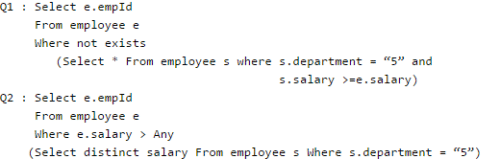Detailed Solution for Test: SQL - Question 8

Let the employee(empId, name, department, salary) have the following instance. empId name department salary ----------------------------------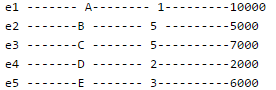Now the actual result should contain empId : e1 , e3 and e5 ( because they have salary greater than anyone employee in the department '5') -------------------------------------------------------- Now Q1 : Note : EXISTS(empty set) gives FALSE, and NOT EXISTS(empty set) gives TRUE.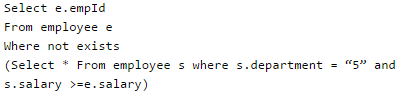Q1 will result only empId e1. --------------------------------------------------------- whereas Q2 :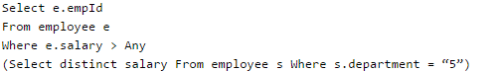Q2 will result empId e1, e3 and e5. -------------------------------------------------------- Hence Q2 is the correct query.

Test: SQL - Question 9

Given the following statements: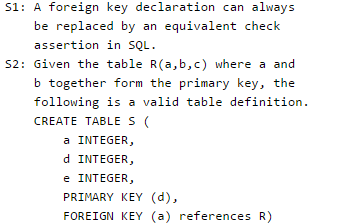Q. Which one of the following statements is CORRECT?

Detailed Solution for Test: SQL - Question 9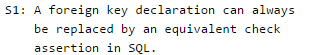False: Check assertions are not sufficient to replace foreign key. Foreign key declaration may have cascade delete which is not possible by just check insertion.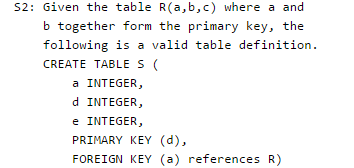False: Foreign key in one table should uniquely identifies a row of other table. In above table definition, table S has a foreign key that refers to field 'a' of R. The field 'a' in table S doesn't uniquely identify a row in table R.

Test: SQL - Question 10

Given the following schema:

employees(emp-id, first-name, last-name, hire-date, dept-id, salary) departments(dept-id, dept-name, manager-id, location-id)

You want to display the last names and hire dates of all latest hires in their respective departments in the location ID 1700. You issue the following query: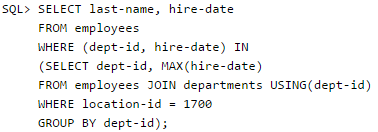Q. What is the outcome?

Detailed Solution for Test: SQL - Question 10

The given query uses below inner query.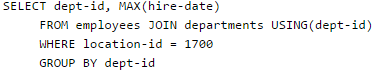The inner query produces last max hire-date in every department located at location id 1700. The outer query simply picks all pairs of inner query. Therefore, the query produces correct result.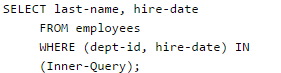Test: SQL - Question 11

SQL allows tuples in relations, and correspondingly defines the multiplicity of tuples in the result of joins. Which one of the following queries always gives the same answer as the nested query shown below:

select * from R where a in (select S.a from S)

Detailed Solution for Test: SQL - Question 11

The solution of this question lies in the data set(tuples) of Relations R and S we define. If we miss some case then we may get wrong answer. Let's say, Relation R(BCA) with attributes B, C and A contains the following tuples.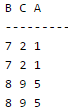And Relation S(AMN) with attributes A, M, and N contains the following tuples.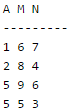Now ,the original Query will give result as: "select * from R where a in (select S.a from S) " - The query asks to display every tuple of Relation R where R.a is present in the complete set S.a.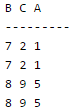Option A query will result in : "select R.* from R, S where R.a=S.a"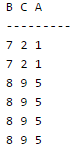Option B query will result in : " select distinct R.* from R,S where R.a=S.a"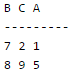----------------------------------------------------------------------------------------------------------- Option C query will result in : "select R.* from R,(select distinct a from S) as S1 where R.a=S1.a" B C A --------- 7 2 1 7 2 1 8 9 5 8 9 5 ----------------------------------------------------------------------------------------------------------- Option D query will result in : NULL set "select R.* from R,S where R.a=S.a and is unique R" ---------------------------------------------------------------------------------------------------------- Hence option C query matches the original result set. Note : As mentioned earlier, we should take those data sets which can show us the difference in different queries. Suppose in R if you don't put identical tuples then you will get wrong answers. (Try this yourself, this is left as an exercise for you).

Test: SQL - Question 12

Consider the following relational schema:

employee(empId, empName, empDept)
customer(custId, custName, salesRepId, rating)

salesRepId is a foreign key referring to empId of the employee relation. Assume that each employee makes a sale to at least one customer. What does the following query return?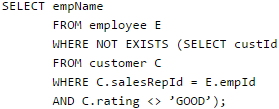Detailed Solution for Test: SQL - Question 12

If any employee has received rating other than 'good' from some customer, then there will be some rows returned by the inner query.
And not exists will return false so that employee won't be printed only those employees which have got rating good from all their customers will be printed.

Test: SQL - Question 13

The statement that is executed automatically by the system as a side effect of the modification of the database is

Test: SQL - Question 14

Which of the following command is used to delete a table in SQL?

Detailed Solution for Test: SQL - Question 14

drop is used to delete a table completely

Test: SQL - Question 15

Consider the relation account (customer, balance) where customer is a primary key and there are no null values. We would like to rank customers according to decreasing balance. The customer with the largest balance gets rank 1. ties are not broke but ranks are skipped: if exactly two customers have the largest balance they each get rank 1 and rank 2 is not assigned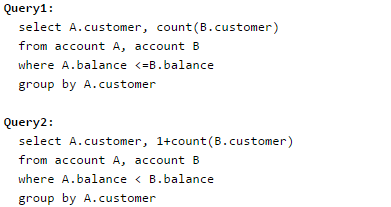Consider these statements about Query1 and Query2.

1. Query1 will produce the same row set as Query2 for some but not all databases.
2. Both Query1 and Query2 are correct implementation of the specification
3. Query1 is a correct implementation of the specification but Query2 is not
4. Neither Query1 nor Query2 is a correct implementation of the specification
5. Assigning rank with a pure relational query takes less time than scanning in decreasing balance order assigning ranks using ODBC.

Q. Which two of the above statements are correct?

Detailed Solution for Test: SQL - Question 15

Query 1 and Query 2 will give the same result if all the customers have distinct balance. So, for some databases, the result of query 1 and query 2 will be same.
Now, let us consider a fact that all the entries in the database have the same value for balance. Ideally, all the customers should have rank 1, but both the queries will give all the customers a rank equal to the number of customers in the database. So, both the queries do not give us the required output.
Thus, C is the correct choice.
Please comment below if you find anything wrong in the above post.

Test: SQL - Question 16

Consider the relation "enrolled(student, course)" in which (student, course) is the primary key, and the relation "paid(student, amount)" where student is the primary key. Assume no null values and no foreign keys or integrity constraints. Given the following four queries: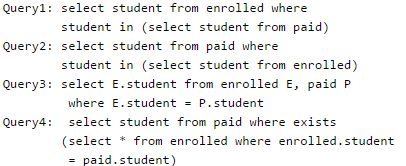Q. Which one of the following statements is correct?

Test: SQL - Question 17

The following table has two attributes A and C where A is the primary key and C is the foreign key referencing A with on-delete cascade.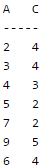The set of all tuples that must be additionally deleted to preserve referential integrity when the tuple (2,4) is deleted is:

Test: SQL - Question 18

The relation book (title, price) contains the titles and prices of different books. Assuming that no two books have the same price, what does the following SQL query list?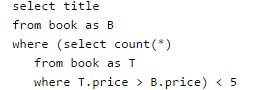Test: SQL - Question 19

Consider the following relation schema pertaining to a students database:

Enroll (rollno, courseno, coursename)

where the primary keys are shown underlined. The number of tuples in the Student and Enroll tables are 120 and 8 respectively. What are the maximum and minimum number of tuples that can be present in (Student * Enroll), where '*' denotes natural join ?

Detailed Solution for Test: SQL - Question 19

The result of the natural join is the set of all combinations of tuples in R and S that are equal on their common attribute names. What is the maximum possible number of tuples? The result of natural join becomes equal to the Cartesian product when there are no common attributes. The given tables have a common attribute, so the result of natural join cannot have more than the number of tuples in larger table.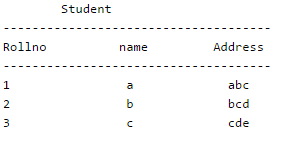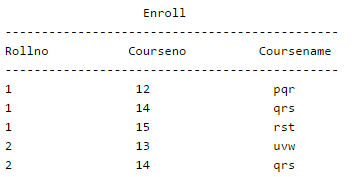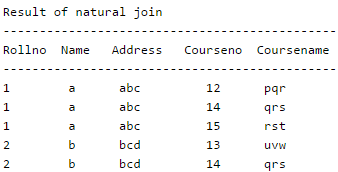What is the maximum possible number of tuples? It might be possible that there is no rollnumber common. In that case, the number of tupples would be 0.

Test: SQL - Question 20

The employee information in a company is stored in the relation

Employee (name, sex, salary, deptName)

Consider the following SQL query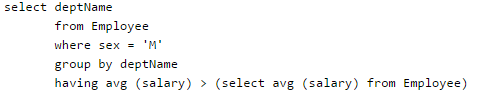It returns the names of the department in which

Detailed Solution for Test: SQL - Question 20

In this SQL query, we have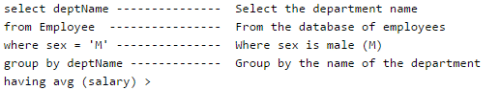(select avg (salary) from Employee) ----- Having the average salary greater than the average salary of all employees in the organization.

So, this query would return the name of all departments in which the average salary of male employees is greater than the average salary of all employees in the company. Hence, D is the correct choice.  Please comment below if you find anything wrong in the above post.

Test: SQL - Question 21

Consider the following SQL query

select distinct al, a2,........., an
from r1, r2,........, rm
where P

For an arbitrary predicate P, this query is equivalent to which of the following relational algebra expressions ?

Detailed Solution for Test: SQL - Question 21

Cross product (x) combines the tuples of one relation with all the tuples of the other relation. Thus, tuples of relation r1, r2 …. rn are combined.

Select operator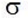is used to select resultant tuples.

Projection operator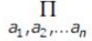is used to select a subset of attributes from the resultant tuples by specifying the names of the attributes. So attributes a1, a2, an are projected from the resultant tuples.

Thus, option (A) is correct.

Please comment below if you find anything wrong in the above post.

Test: SQL - Question 22

Consider the set of relations shown below and the SQL query that follows.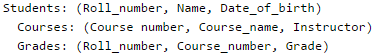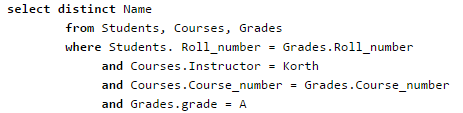Q. Which of the following sets is computed by the above query?

Detailed Solution for Test: SQL - Question 22

The query gives the name of all the students who have scored "A" grade in any of the courses that are taught by Korth. So, C is the correct choice.

Please comment below if you find anything wrong in the above post.

Test: SQL - Question 23

Given relations r(w, x) and s(y, z), the result of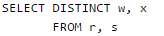is guaranteed to be same as r, provided

Test: SQL - Question 24

In SQL, relations can contain null values, and comparisons with null values are treated as unknown. Suppose all comparisons with a null value are treated as false. Which of the following pairs is not equivalent?

Detailed Solution for Test: SQL - Question 24

For all values smaller than 5, x < 5 will always be true but x = 5 will be false.

Test: SQL - Question 25

Consider the following three table to store student enrollements in different courses.

Student(EnrollNo, Name)
Course(CourseID, Name)
EnrollMents(EnrollNo, CourseID)

Q. What does the following query do?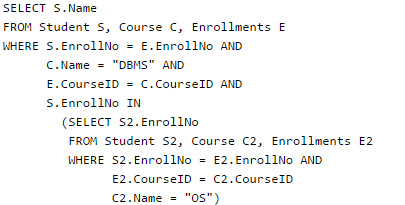Detailed Solution for Test: SQL - Question 25

Background Reading: The above query is an example of nested query i.e. query within a query. Firstly the inner query is solved and then the outer one depending on the result of the inner query.

• WHERE IN returns values that matches values in a list or subquery.
• WHERE IN is a shorthand for multiple OR conditions.

Here, firstly the inner query is solved. It returns all the Enrollment
Numbers (SELECT S2.EnrollNo) of students where the students’ enrollment
number matches with the enrollment number of the courses
(WHERE S2.EnrollNo = E2.EnrollNo) which have the course IDs whose Course
Name is “OS” (E2.CourseID = C2.CourseID and C2.Name = “OS”).

Hence all the enrollment IDs are filtered out for the students who are enrolled for the “OS” course.

The outer query works similarly and filters out all the all tuples where the Students Enrollment Number matches with the Enrollment Number where the course ID’s are for the course names “DBMS”
(S.EnrollNo = E.EnrollNo AND C.Name =”DBMS” AND E.CourseID = C.CourseId) and additionally matches with the ones that are returned by the inner query i.e. Enrollment Number of students who are enrolled for the course “OS”.

Hence the above queries returns names of all students (SELECT S.Name) who have enrolled for both courses “DBMS” and “OS”. Hence option (B).

Test: SQL - Question 26

Consider the following Employee table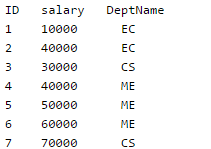How many rows are there in the result of following query?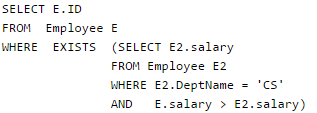Detailed Solution for Test: SQL - Question 26

Background:

1. WHERE EXISTS tests for the existence of any records in a subquery.
2. EXISTS returns true if the subquery returns one or more records.
3. EXISTS is commonly used with correlated subqueries.

Here in the above question, there is a correlated subquery because the subquery references the enclosing query (relation Employee renamed as E) The subquery (SELECT E2.salary FROM Employee E2 WHERE E2.DeptName = 'CS') Filters out E2 relation as (all tuples where DeptName is CS and the respective salaries) Now the correlated query works as follows: SELECT E.ID FROM Employee E WHERE EXISTS (SELECT E2.salaryFROM Employee E2 WHERE E2.DeptName = 'CS' AND E.salary > E2.salary) It takes one tuple from the Employee Relation and displays its ID if the WHERE EXISTS returns true i.e. the subquery returns one or more records. This happens in the case when the tuple from the Employee Relation E has the value of the salary attribute greater than any one of the values of the salary attribute filtered out above. So tuples filtered out would be all the tuples that have their salary attribute value greater than the salary values of at least one from the E2 relation (3000 and 7000). ID salary DeptName 2 40000 EC 4 40000 ME 5 50000 ME 6 60000 ME 7 70000 CS Finally it displays their ID’s and the output would be: 2 4 5 6 7 Hence option (C) 5 rows.

Test: SQL - Question 27

Select operation in SQL is equivalent to

Detailed Solution for Test: SQL - Question 27

Select operation is equivalent to the projection operation in relational algebra, except that select in SQL retains duplicates and on the contrary projection removes the duplicates.

Test: SQL - Question 28

Consider the following relations: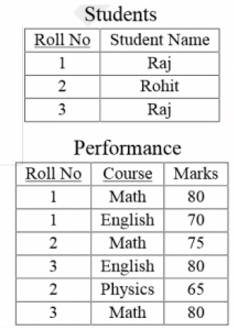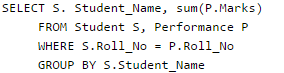The number of rows that will be returned by the SQL query is _________

Detailed Solution for Test: SQL - Question 28

Below is result of given query. Note that there are only two student names and query prints sum(P.Marks) for every student.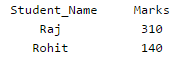Test: SQL - Question 29

Consider the following relation

Which of the following options will be needed at the end of the SQL query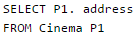Q. Such that it always finds the addresses of theaters with maximum capacity?

Detailed Solution for Test: SQL - Question 29

When the ALL condition is followed by a list, the optimizer expands the initial condition to all elements of the list and strings them together with AND operators. When the ANY condition is followed by a list, the optimizer expands the initial condition to all elements of the list and strings them together with OR operators, as shown below.

Test: SQL - Question 30

A company maintains records of sales made by its salespersons and pays them commission based on each individual's total sales made in a year. This data is maintained in a table with following schema:

salesinfo = (salespersonid, totalsales, commission)

In a certain year, due to better business results, the company decides to further reward its salespersons by enhancing the commission paid to them as per the following formula:

If commission < = 50000, enhance it by 2% If 50000 < commission < = 100000, enhance it by 4% If commission > 100000, enhance it by 6%

The IT staff has written three different SQL scripts to calculate enhancement for each slab, each of these scripts is to run as a separate transaction as follows: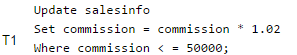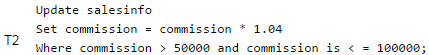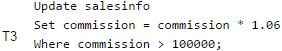Q. Which of the following options of running these transactions will update the commission of all salespersons correctly

Detailed Solution for Test: SQL - Question 30

T3 followed by T2 followed by T1  If this sequence is not followed then it may happen that Officer of one slab get benefited twice. Say an officer is having commission as 99,999,He must be get updated commision according to T2.But then 99,999*1.04=1,03998 and he again becomes eligible for next slab of commision. T3 followed by T2 followed by T1 will be check all border cases like above.So Answer is D

## GATE Computer Science Engineering(CSE) 2023 Mock Test Series

150 docs|215 tests
 Use Code STAYHOME200 and get INR 200 additional OFF Use Coupon Code